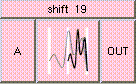SHIFTTHE BOX'S MAIN HELP: The result is (A+1)/2. This shifts the range of A from [-1,1] to [0,1]. Inputs should lie in the range [-1,1]. A (input): The value to be shifted. Inputs should lie in the range [-1,1]. OUT (output): The result is (A+1)/2. This shifts the range of A from [-1,1] to [0,1]. Inputs should lie in the range [-1,1].

<-- BACK TO Math